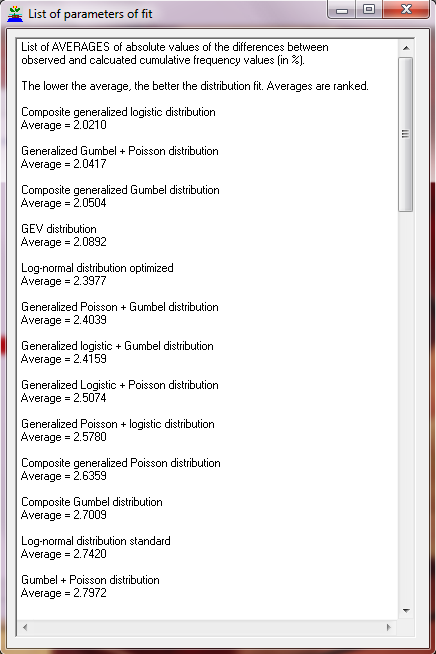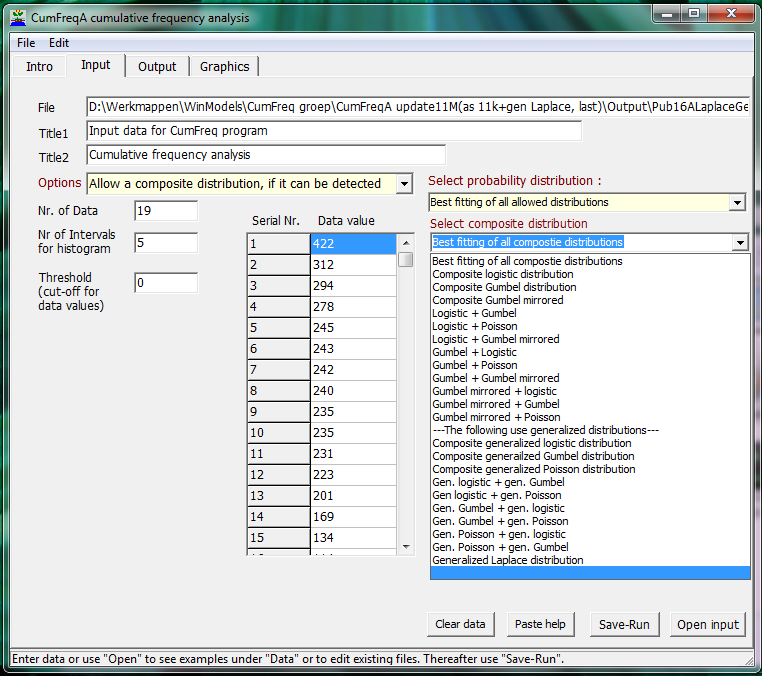CumFreq:   Cumulative frequency analysis with probability distribution fitting Free download of software

CumFreq

Software
& models

General articles
& manuals

Artículos
(in Spanish,
en Español)

Published
reports & cases

Particular
reports & cases

FAQ's

Update
record

Home
page

 Hints: CumFreq can create histograms and probability density functions as illustrated on the density page. An amplified version of CumFreq named CumFreqA can be seen on: composite distributions. This version contains extra facilities regarding composite probability distributions. These are not often found with an exception of the Laplace distribution.           The versatility of generalization makes it possible, for example, to fit approximately normally distributed data sets to a large number of different probability distributions, see multple fit, while negatively skewed distributions can be fitted to square normal and mirrored Gumbel distributions, as in left skew, and finally the various possibilities of curved regression are show in curvatures.Introduction screen to CumFreq program (model). The CumFreq calculator models the cumulative (non-exceedance) frequency distribution and fits it to a probability distribution. It uses, amongst other, the normal, logistic, exponential and Gumbel distributions. For distribution fitting, the CumFreq calculator app linearizes the probability distribution.For a list of linearizations, based on logarithmic and other transformations, see: transformationsInput tabsheet The input tabsheet shows the probability distributions that are being used in the CumFreq calculator model to fit the data to. In this application program one can select "best of all" or indicate a preference. The number of intervals needed to prepare the histogram can be entered, as well as a cut-off (threshold) velue.Cumulative distribution fitting Probability distribution fitting is based on plotting positions (the observed data). A 90% confidence interval of the fitted probability distribution is shown. It is a specialty of the CumFreq calculator model calculator to apply "generalized" distributions, which, in this application program, makes them fit better than the standard ones. They are based on an exponential transformation of the data to obtain a closer fit. The curve shown is also called cumulative distribution function (PDF).Histogram of fitted distribution + density function The histogram provides the observed and calculated frequencies by interval. The histogram gives an impression of the symmetry of the probability distribution and whether it is skew to the left or to the right. The probability density function (PDF) is shown as the product of density and lenhth of the intervals.Probability distributions ranked by goodness of fit CumFreq provides the option to produce a list of probability distributions ranked by goodness of fit.Calculator CumFreq provides the option to calculate probabilities of data values and vice versa. The same holds for return periods. In addition confidence intervals are given.Composite probabilty distributions. The amplified CumFreq calculator (CumFreqA) provides the possibility to select a composite probability distribution (like the Laplace distribution) or to find the best fitting of all.